• 如果半正定的话，那么是≥0，其物理意义就是发送信号的能量大于等于0，即可以部分天线不发送。 奇异矩阵和非奇异矩阵 如果A（n×n）为奇异矩阵（singular matrix），那么矩阵A的秩，Rank(A)。 如果A（n×n）为非...
判断矩阵正定三个条件

1. 顺序主子式都>0
2. 特征值都>0
3. 矩阵的正惯性指数=n（矩阵的阶数）
如果半正定的话，那么是≥0，其物理意义就是发送信号的能量大于等于0，即可以部分天线不发送。
奇异矩阵和非奇异矩阵
如果A（n×n）为奇异矩阵（singular matrix），那么矩阵A的秩，Rank(A)＜n。
如果A（n×n）为非奇异矩阵（nonsingular matrix），那么矩阵A满秩，Rank(A)=n。
其中矩阵的秩的意思是，纵秩为其矩阵的线性无关的纵列的最大数目，行秩为矩阵的线性无关的横行的最大数目。
矩阵的迹
矩阵X的主对角线上所有元素之和称为X的迹，记为tr(X)，即tr(X)=Σx_ii。
在对角矩阵中，实际上就是对角线上值的和。如果svd分解完的矩阵，中间的矩阵正定，就是其能量。在MIMO中，其矩阵的迹是能量。
正惯性指数
正惯性指数是矩阵二次型的标准型中平方项系数的正负个数。
二次型的矩阵

f(x,y)=x²+4xy+5y²f(x,y,z)=2x²+y²+4xz+yzf(x1,x2,x3,x4)=x1x2+x2x3+x2x4
不是二次型的矩阵

f(x,y)=x²+y²+5f(x,y)=2x²-y²+2x

二次型的标准型
只含有平方项的二次型称为二次型的标准型（或法式），即

f=k1y1²+k2y2²+ ... +knyn²
例如

f(x1,x2,x3)=x1²+4x2²+4x3²，即是二次型的标准型。


展开全文• 先说结论：协方差矩阵都是半正定（PSD）协方差矩阵正定（PD）需要条件，要求满多元高斯分布协方差矩阵未必是正定（PD）。首先，证明协方差矩阵半正定（PSD）。比较常见证明，方法很多。举个例子2. 然后，...先说结论：协方差矩阵都是半正定（PSD）的协方差矩阵正定（PD）需要条件，要求满秩多元高斯分布的协方差矩阵未必是正定（PD）的。首先，证明协方差矩阵半正定（PSD）。比较常见的证明，方法很多。举个例子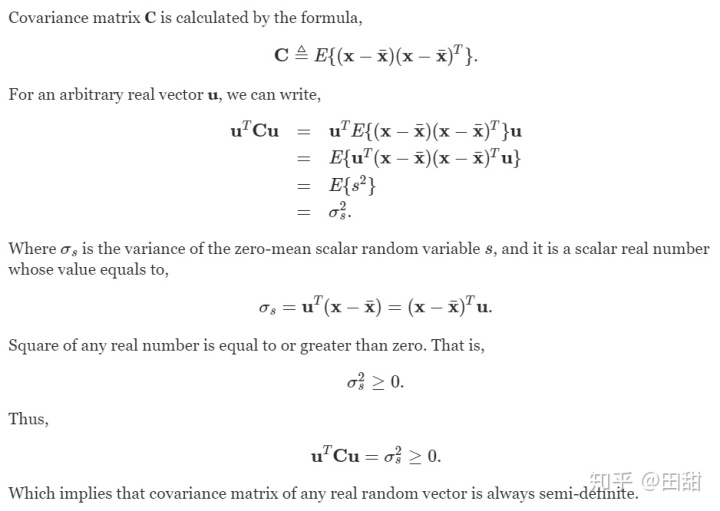2. 然后，证明正定（PD）的条件是满秩。因为已经证明半正定， 即, 要证明正定，即证明“=”不成立。可以用反证法：(知乎输入法不熟)因为u是非零向量，那么u'z=0有非零解u意味着z不满秩, 即等号成立z不满秩。反之，如果z满秩，则=不能成立，即半正定升级为正定。3. 多元高斯分布的协方差矩阵Andrew Ng 在 CS229 Leacture Notes, Part X,  Factor Analysis 这一节也有讨论。如果sample size n 远小于维度p, 协方差矩阵的逆的确不存在。此时我们通过给sigma加一些约束来改造它。方法1： fit一个diagonal的sigma; 即各维度之间独立，对角线为各维度的variance的均值.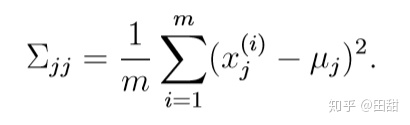方法2：sigma不仅是diagonal的，而且所有的对角线元素都相同，即; 将所有m个sample的variance取平均：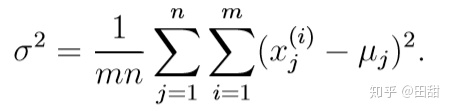最后，引用一下@Vicktore 关于高斯分布的讨论：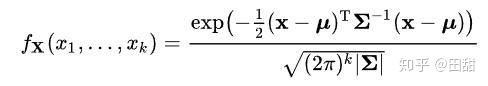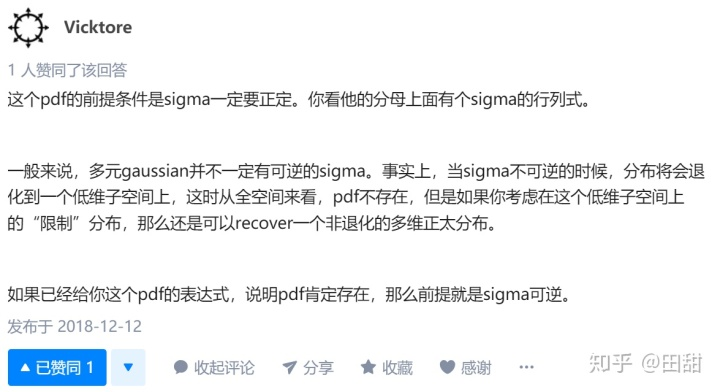展开全文• 在线性系统理论中，如果系统是能控的，...[图片说明](https://img-ask.csdn.net/upload/202002/01/1580564165_539123.jpg)中，我先是用卡尔曼判据判断为可控，可是它的格莱姆矩阵的特征值总是存在负值，这是为什么？中文分词 全文检索 深度学习 神经网络
• 文章目录可逆表示正定可逆实对称表示正定实对称表示半正定正定表示正定半正定表示半正定正定与主子式一些重要xiao结论列满表示正定行满表示半正定上三角表示正定 可逆表示正定 实对称A为正定⇔\Leftrightarrow...
文章目录可逆表示正定可逆实对称表示正定实对称表示半正定正定表示正定半正定表示半正定正定与主子式一些重要的xiao结论列满秩表示正定行满秩表示半正定上三角表示正定
可逆表示正定
实对称A为正定$\Leftrightarrow$存在实可逆C使得$A=C'C$
可逆实对称表示正定
实对称A为正定$\Leftrightarrow$存在可逆实对称C使得$A=C^2$
实对称表示半正定
实对称A为半正定$\Leftrightarrow$存在实对称C使得$A=C^2$
注意！这里不要求C是可逆啦！
正定表示正定
A为正定$\Leftrightarrow$存在唯一正定C使得$A=C^2$
半正定表示半正定
A为半正定$\Leftrightarrow$存在唯一半正定C使得$A=C^2$
正定与主子式
实对称A为正定$\Leftrightarrow A$的主子式都$>0$
一些重要的xiao结论

A正定，B半正定$\Rightarrow$ A+B正定
A正定，B正定$\Rightarrow$ A+B正定
A正定$\Rightarrow$ $A^{-1},A^*$都是正定

列满秩表示正定
实对称A正定$\Leftrightarrow$存在$m×n$列满秩矩阵P,使得$A=P'P$
行满秩表示半正定
实对称A半正定$\Leftrightarrow$存在$r×n$列满秩矩阵Q,使得$A=Q'Q$
上三角表示正定
实对称A正定$\Leftrightarrow$存在上三角B,且B的主对角元都>0,使得$A=B'B$


展开全文• 文章目录可逆表示正定证明可逆实对称表示正定证明实对称表示半正定正定表示正定证明半正定表示半正定正定与主子式证明一些重要xiao结论列满表示正定证明行满表示半正定证明上三角表示正定证明 可逆表示正定 实...
文章目录可逆表示正定证明可逆实对称表示正定证明实对称表示半正定正定表示正定证明半正定表示半正定正定与主子式证明一些重要的xiao结论列满秩表示正定证明行满秩表示半正定证明上三角表示正定证明
可逆表示正定
实对称A为正定$\Leftrightarrow$存在实可逆C使得$A=C'C$
证明

实对称A为正定
$\Leftrightarrow$ $A\simeq I$
$\Leftrightarrow$ $\exist实可逆C使得$ $A=C'IC$

可逆实对称表示正定
实对称A为正定$\Leftrightarrow$存在可逆实对称C使得$A=C^2$
证明

必要性：由于A是正定实对称，则存在正交矩阵$T$使得$A=T'DT$ $D=diag\{\lambda_1,...,\lambda_n\}$ $=diag\{\sqrt{\lambda_1},...,\sqrt{\lambda_n}\}^2=D_1^2$
于是$A=T'D_1TT'D_1T=C^2$,易得C是实对称

实对称表示半正定
实对称A为半正定$\Leftrightarrow$存在实对称C使得$A=C^2$
注意！这里不要求C是可逆啦！
正定表示正定
A为正定$\Leftrightarrow$存在唯一正定C使得$A=C^2$
证明
这里的证明思路是：通过$T'D^2T=T_1'D^2T_1$来证明：$T'DT=T_1'DT_1$

正定：由上可逆实对称表示正定得，C的特征值都是正数，所以这个C就是正定矩阵
下面证明这样的C是唯一的，骚操作来啦

反证，假设还有个$C_1$满足要求
设$C_1$的全部特征值为$v_1,...,v_n$,则A的全部特征值为$v_1^2,...,v_n^2$
设$C$的全部特征值为$\mu_1,...,\mu_n$,则A的全部特征值为$\mu_1^2,...,\mu_n^2$
所以适当调换位置后可以得到$\mu_i^2=v_i^2$又∵$\mu_i,v_i>0$∴$\mu_i=v_i$
又因为$C,C_1$均为实对称矩阵，所以存在正交矩阵$T,T_1$满足$C=T^{-1}diag\{\mu_1,...,\mu_n\}T$ $C_1=T_1^{-1}diag\{v_1,...,v_n\}T_1$下面证明：$C=C_1$
由于$C^2=C_1^2$，所以有$T^{-1}diag\{\mu_1^2,...,\mu_n^2\}T=T_1^{-1}diag\{\mu_1^2,...,\mu_n^2\}T_1$ $\Leftrightarrow$ $T_1T^{-1}diag\{\mu_1^2,...,\mu_n^2\}=diag\{\mu_1^2,...,\mu_n^2\}T_1T^{-1}\tag{1}$
记$T_1T^{-1}=(t_{ij})$,比较(1)式两边的$(i,j)$元素，得$t_{ij}\mu_j^2=t_{ij}\mu_i^2$分两种情况讨论

$t_{ij}\ne0$

则$\mu_i=\mu_j$，可得，所有$\mu_i$都相等，所以就有$t_{ij}\mu_j=t_{ij}\mu_i\tag{2}$

$t_{ij}=0$

如此的话，显然（2）式也成立

所以就有$T_1T^{-1}diag\{\mu_1,...,\mu_n\}=diag\{\mu_1,...,\mu_n\}T_1T^{-1}\tag{1}$ $\Leftrightarrow$ $C=C_1$

注意！！
这里证明的是$C_1=C$，还有一种思路是证$T=T_1$，虽然这样的证法理由看似直观，因为对角矩阵都一样了，那正交矩阵肯定都一样了呀,但是这是不对的，这是一个大坑！！因为可以用不同的正交矩阵得到一样的对角矩阵！还记得T是怎么来的吗？特征向量标准正交化，完全可选不同的特征向量呀！
半正定表示半正定
A为半正定$\Leftrightarrow$存在唯一半正定C使得$A=C^2$
正定与主子式
实对称A为正定$\Leftrightarrow A$的主子式都$>0$
证明
之前和主子式有关的定理是：A正定$\Leftrightarrow A$的所有顺序主子式都>0
这里相当于拓展了一下结论

由之前的可逆表正定得，存在可逆矩阵C满足$A=C'C$
则$A\begin{pmatrix}i_1&i_2&...&i_k\\i_1&i_2&...&i_k\end{pmatrix}=C'C\begin{pmatrix}i_1&i_2&...&i_k\\i_1&i_2&...&i_k\end{pmatrix}$ $=\sum C'\begin{pmatrix}i_1&i_2&...&i_k\\v_1&v_2&...&v_k\end{pmatrix}C\begin{pmatrix}v_1&v_2&...&v_k\\i_1&i_2&...&i_k\end{pmatrix}$ $=\sum C^2\begin{pmatrix}v_1&v_2&...&v_k\\i_1&i_2&...&i_k\end{pmatrix}$
由于C可逆，所以C的第$i_1,i_2,...,i_k$列组成的子矩阵$C_1$是列满秩矩阵，故肯定存在一个不为0的k阶子式使得$\sum C^2> 0$

一些重要的xiao结论

A正定，B半正定$\Rightarrow$ A+B正定
A正定，B正定$\Rightarrow$ A+B正定
A正定$\Rightarrow$ $A^{-1},A^*$都是正定

列满秩表示正定
实对称A正定$\Leftrightarrow$存在$m×n$列满秩矩阵P,使得$A=P'P$
证明
啊这里好简单的！我还想那么复杂真的是太傻啦

A正定$\Rightarrow\exist$实可逆C，使得$A=C'C$
只要令$P=\begin{pmatrix}C\\0\end{pmatrix}_{m×n}$,则有$(C',0)\begin{pmatrix}C\\0\end{pmatrix}=A$

行满秩表示半正定
实对称A半正定$\Leftrightarrow$存在$r×n$列满秩矩阵Q,使得$A=Q'Q$
证明

假设$rank(A)=r，$则$A\simeq I_r$(典型错误写法！！应该是$A\simeq\begin{pmatrix}I_r&0\\0&0\end{pmatrix}$)所以$\exist可逆C$满足$C'\begin{pmatrix}I_r&0\\0&0\end{pmatrix}C=A$
∴只要让$C=\begin{pmatrix}Q_{r×n}\\H\end{pmatrix}$有$(Q',H')\begin{pmatrix}I_r&0\\0&0\end{pmatrix}\begin{pmatrix}Q_{r×n}\\H\end{pmatrix}=Q'Q$Q当然是行满秩啦！C可是可逆矩阵

上三角表示正定
实对称A正定$\Leftrightarrow$存在上三角B,且B的主对角元都>0,使得$A=B'B$
证明

必要性：若实对称A正定$\Rightarrow\exist$可逆矩阵C，有$A=C'C$

之前还有个结论，可逆矩阵可以表示为$正交矩阵T×主对角元都>0的上三角B$
所以$C=TB$,$A=B'T'TB=B'B$

充分性：若$A=B'B$,首先A肯定是对称的

这里用的是正定矩阵的等定义来证：对$\forall\alpha\ne0\in R^n$，有$B\alpha\ne0(B可逆)$ $\alpha'B'B\alpha=(B\alpha)'B\alpha>0$guA正定


展开全文• 对于实二次型其中A是实对称，下列条件等价： 正定 （1）是正定. ...半正定的 （1）是半正定的. （2）它正惯性指数p与r相等. （3）有可逆实矩阵C，使得其中 （4）有实矩阵C使得A=C'C. （5...
• Laplacian矩阵的性质： 一是为对称阵 二是存在一个为零的特征值（为|V|-1） 三是一个半正定矩阵
• 半正定矩阵3. 可逆矩阵4. 伴随矩阵5. 对角化6. 正交矩阵7. 奇异矩阵8. 相似矩阵9. 矩阵秩的性质10. 参考 1. 正定矩阵 （若A为正定矩阵，则-A为负定矩阵。要判断一个矩阵H是否为负定矩阵，只需判断-H是否为正定矩阵...
• 这就是对矩阵 奇异值分解，示意图如下所示：奇异值分解示意图对于奇异值定义是这样：假设矩阵 ， ，由线性代数知识可知 是半正定矩阵，可令矩阵 特征值为 。称 为矩阵 奇异值。2. 矩阵奇异值分解过程假设...
• 一个矩阵A(方阵)半正定，且它每个特征值大于或等于0，则A为奇异矩阵。 一个矩阵A(方阵)正定,且它每个特征值都大于0，为奇异矩阵。 一个方阵非奇异当且仅当它代表线性变换是个自同构，为奇异矩阵。 非奇异矩阵...线性代数
• 向量乘法2.3 矩阵-矩阵乘法3 运算和属性3.1 单位矩阵和对角矩阵3.2 转置3.3 对称矩阵3.4 矩阵的迹3.5 范数3.6 线性相关性和3.7 方阵的逆3.8 正交阵3.9 矩阵的值域和零空间3.10 行列式3.11 二次型和半正定矩阵3.12 ...机器学习 线性代数 人工智能
• 目录第0章 综述与杂叙0.0 引言0.1 向量空间0.2 矩阵0.3 行列式...对称矩阵以及相合第5章 向量的范数与矩阵的范数第6章 特征值的位置与摄动第7章 正定矩阵以及半正定矩阵第8章 正的矩阵与非负的矩阵 第0章 综述与杂...
• //特征值，行列式，，对称矩阵，单位矩阵，正定半正定，雅可比等等！！ 正交矩阵： 如果：AA'=E（E为单位矩阵，A'表示“矩阵A转置矩阵”。）或A′A=E，则n阶实矩阵A称为正交矩阵， 若A为正交阵，则满足以下...
• 所以协方差矩阵满足实对称矩阵和半正定矩阵的性质。 记Σ\SigmaΣ为协方差矩阵。 Σ\SigmaΣ是满的，也就是∣Σ∣|\Sigma|∣Σ∣（行列式）不为0。 Σ\SigmaΣ是半正定的，所以∣Σ∣|\Sigma|∣Σ∣是大于0。 Σ\...
• ## 矩阵

2020-11-16 16:12:28
SVD奇异值分解 A’ A => 奇异值 PCA降维，选择特征值最大的维度 各方向方差，方差占得比例 ...矩阵的秩 矩阵等价 系数矩阵 正交矩阵 特征值和特征矩阵 对角化 正定矩阵半正定 QR分解 求特征值 向量求导 ...机器学习
• 将图像域 中点 x上的对称半正定矩阵M1=M1(x)定义为： 则M1的秩为1。对于图像的每一个像素，都可以计算得出该矩阵。 选择权重矩阵W（通常是高斯滤波器），可以得到卷积： 这个卷积的目的是得到M1在周围像素上...
• 1.8 求矩阵的秩29 1.9 对称正定矩阵的乔里斯基分解与行列式求值33 1.10 矩阵的三角分解36 1.11 一般实矩阵的QR分解41 1.12 一般实矩阵的奇异值分解46 1.13 求广义逆的奇异值分解法61 第2章 矩阵特征值与特征向量的...
• 我们把图像域中点x上的对称半正定矩阵MI=MI(x)定义为： 其中∇I为包含导数Ix和Iy的图像梯度。由于该定义，MI的秩为1，特征值为λ1=|∇I|2和λ2=0。现在对于图像的每个像素，我们可以计算出该矩阵。 选择权重矩阵W...
• ## SVD模型裁剪 TDNN-f

千次阅读 2019-02-27 13:02:25
Simi-Orthogonal Low-Rank Matrix Factorization for Deep Neural Networks 半正定秩矩阵分解DNN 摘要： TDNN又被称为1维CNN(1dCNNS)。本文提出TDNN-F，结构与经过SVD分解TDNN相同。但TDNN-F训练开始于随机...
• SIFT地理特征匹配（python）一、Harris角点检测二、SIFT图像匹配1....我们把图像域中点x上的对称半正定矩阵MI=MI(x)定义为： 其中∇I为包含导数Ix和Iy的图像梯度。由于该定义，MI的秩为1，特征值为λ1=python 计算机视觉
• 文章目录1.Harris角点检测1.1在图像间寻找对应点2.sift描述子2.1兴趣点2.2描述子...我们把图像域中点x上的对称半正定矩阵MI=MI(x)定义为： 其中∇I为包含导数Ix和Iy的图像梯度。由于该定义，MI的秩为1，特征值为λ1=|python 计算机视觉
• 第6章通过最小化增广多维尺度矩阵的秩，利用交替方向乘子法求解该矩阵及其辅助参数，对获得的低秩矩阵进行特征值分解从而求得椭圆参数。第7章通过选择较好的椭圆点来表示椭圆参数向量，并采用误差的绝对值之和降低野...图像
• 矩阵运算1 1.1 实矩阵相乘1 1.2 复矩阵相乘4 1.3 一般实矩阵求逆8 1.4 一般复矩阵求逆13 1.5 对称正定矩阵的求逆18 1.6 托伯利兹矩阵求逆的特兰持方法21 1.7 求一般行列式的值25 1.8 求矩阵的秩29 1.9 对称正定矩阵...
• （有限存储Hessian矩阵半正定）。其他特征包括使用松弛程序来处理不可行非线性约束、在工作集零空间中使用良态非正交基QP（由稀疏校正LU因子辅助）、QP子问题提前终止以及对缺失梯度有限差分估计。nlp
• ## C++常用算法程序集

热门讨论 2011-03-24 09:15:32
1.8 求矩阵的秩29 1.9 对称正定矩阵的乔里斯基分解与行列式求值33 1.10 矩阵的三角分解36 1.11 一般实矩阵的QR分解41 1.12 一般实矩阵的奇异值分解46 1.13 求广义逆的奇异值分解法61 第2章 矩阵特征值与特征向量的...
• 范例1-8 查找矩阵的马鞍点 19 ∷相关函数：Get_Saddle函数 1.1.9 对角矩阵建立 21 范例1-9 对角矩阵建立 21 ∷相关函数：Store函数 1.1.10 三对角矩阵的建立 22 范例1-10 三对角矩阵的建立 22 ∷相关函数：...
• 范例1-8 查找矩阵的马鞍点 19 ∷相关函数：Get_Saddle函数 1.1.9 对角矩阵建立 21 范例1-9 对角矩阵建立 21 ∷相关函数：Store函数 1.1.10 三对角矩阵的建立 22 范例1-10 三对角矩阵的建立 22 ∷相关函数：...
• 范例1-8 查找矩阵的马鞍点 19 ∷相关函数：Get_Saddle函数 1.1.9 对角矩阵建立 21 范例1-9 对角矩阵建立 21 ∷相关函数：Store函数 1.1.10 三对角矩阵的建立 22 范例1-10 三对角矩阵的建立 22 ∷相关函数：...
• 范例1-8 查找矩阵的马鞍点 19 ∷相关函数：Get_Saddle函数 1.1.9 对角矩阵建立 21 范例1-9 对角矩阵建立 21 ∷相关函数：Store函数 1.1.10 三对角矩阵的建立 22 范例1-10 三对角矩阵的建立 22 ∷相关函数：...